﻿ 基于加权截断Schatten-p范数与改进二阶全变分的矩阵填充 Matrix Completion Based on Weighted Truncated Schatten-p Norm and Improved Second Order Total Variation

Artificial Intelligence and Robotics Research
Vol. 08  No. 01 ( 2019 ), Article ID: 28965 , 12 pages
10.12677/AIRR.2019.81004

Matrix Completion Based on Weighted Truncated Schatten-p Norm and Improved Second Order Total Variation

Gang Chen

School of Mathematics and Statistics, Southwest University, Chongqing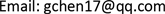Received: Jan. 24th, 2019; accepted: Feb. 13th, 2019; published: Feb. 22nd, 2019ABSTRACT

In recent years, matrix completion problem has attracted researchers’ interest in many practical applications. A lot of matrix completion methods based on low rank matrix recovery theory have been developed. In these methods, only the low rank prior information of matrices is considered. However, in the practical application of matrix completion, the local smooth priori information has not been better utilized, which leads to poor matrix completion effect. To solve the above problems, this paper proposes a matrix completion model based on weighted truncated Schatten-p norm and improved second-order total variation. This model uses weighted truncated Schatten-p norm to constrain the matrix with low rank prior. The smooth prior of the matrix is modeled by the improved second-order total variation norm. Compared with the experimental results of many existing matrix completion methods in text mask image reconstruction, the proposed method has better completion effect.

Keywords:Matrix Completion, Weighted Truncated Schatten-p Norm, Smooth Prior, Improved Second-Order Total Variation1. 引言

$\begin{array}{l}\underset{X}{\mathrm{min}}\text{\hspace{0.17em}}\text{\hspace{0.17em}}\text{\hspace{0.17em}}\text{rank}\left(X\right)\\ \text{s}\text{.t}\text{.}\text{\hspace{0.17em}}\text{\hspace{0.17em}}\text{\hspace{0.17em}}\text{ }{X}_{\Omega }={M}_{\Omega }\end{array}$ (1)

$\begin{array}{l}\underset{X}{\mathrm{min}}\text{\hspace{0.17em}}\text{\hspace{0.17em}}\text{\hspace{0.17em}}{‖X‖}_{\ast }\\ \text{s}\text{.t}\text{.}\text{\hspace{0.17em}}\text{\hspace{0.17em}}\text{\hspace{0.17em}}\text{\hspace{0.17em}}{X}_{\Omega }={M}_{\Omega }\end{array}$ (2)

${‖·‖}_{\omega ,p}={\left({\sum }_{i=1}^{\mathrm{min}\left(m,n\right)}{\omega }_{i}{\sigma }_{i}^{p}\left(·\right)\right)}^{1/p}$$0，并指出该范数可以更精确的逼近秩函数。

2. WTP-MSTVM模型的建立

$\begin{array}{l}\underset{X}{\mathrm{min}}{‖X‖}_{\omega ,r}^{p}+\lambda {‖X‖}_{MSTV}\\ \text{s}\text{.t}\text{.}\text{\hspace{0.17em}}\text{\hspace{0.17em}}\text{\hspace{0.17em}}{X}_{\Omega }={M}_{\Omega },\end{array}$ (3)

2.1. 低秩正则化约束

${‖X‖}_{w,r}^{p}=\underset{i=r}{\overset{\mathrm{min}\left(m,n\right)}{\sum }}{w}_{i}{\sigma }_{i}^{p}\left(X\right)$ (4)

${w}_{i}=\frac{C\sqrt{mn}}{{\sigma }_{i}^{1/p}\left(X\right)+\epsilon }$ (5)

2.2. 改进二阶全变分正则约束

${‖X‖}_{MSTV}=\underset{i=1}{\overset{m}{\sum }}\underset{j=1}{\overset{n}{\sum }}\left({\left({G}_{i,j}^{v}\left(X\right)\right)}^{2}+{\left({G}_{i,j}^{h}\left(X\right)\right)}^{2}\right)$ (6)

${G}_{i,j}^{v}\left(X\right)=\left\{\begin{array}{l}{X}_{i+1,j}-{X}_{i,j}\text{\hspace{0.17em}}\text{\hspace{0.17em}}\text{\hspace{0.17em}}\text{\hspace{0.17em}}\text{\hspace{0.17em}}\text{\hspace{0.17em}}\text{\hspace{0.17em}}\text{\hspace{0.17em}}\text{\hspace{0.17em}}\text{\hspace{0.17em}}\text{\hspace{0.17em}}\text{\hspace{0.17em}}\text{\hspace{0.17em}}\text{\hspace{0.17em}}\text{\hspace{0.17em}}\text{\hspace{0.17em}}\text{\hspace{0.17em}}\text{\hspace{0.17em}}\text{\hspace{0.17em}}i=1\\ {X}_{i-1,j}+{X}_{i+1,j}-2{X}_{i,j}\text{\hspace{0.17em}}\text{\hspace{0.17em}}\text{\hspace{0.17em}}\text{\hspace{0.17em}}\text{\hspace{0.17em}}1 (7)

${G}_{i,j}^{h}\left(X\right)=\left\{\begin{array}{l}{X}_{i,j+1}-{X}_{i,j}\text{\hspace{0.17em}}\text{\hspace{0.17em}}\text{\hspace{0.17em}}\text{\hspace{0.17em}}\text{\hspace{0.17em}}\text{\hspace{0.17em}}\text{\hspace{0.17em}}\text{\hspace{0.17em}}\text{\hspace{0.17em}}\text{\hspace{0.17em}}\text{\hspace{0.17em}}\text{\hspace{0.17em}}\text{\hspace{0.17em}}\text{\hspace{0.17em}}\text{\hspace{0.17em}}\text{\hspace{0.17em}}\text{\hspace{0.17em}}\text{\hspace{0.17em}}\text{\hspace{0.17em}}\text{\hspace{0.17em}}\text{\hspace{0.17em}}j=1\\ {X}_{i,j-1}+{X}_{i,j+1}-2{X}_{i,j}\text{\hspace{0.17em}}\text{\hspace{0.17em}}\text{\hspace{0.17em}}\text{\hspace{0.17em}}\text{\hspace{0.17em}}\text{\hspace{0.17em}}1 (8)

${‖X‖}_{MSTV}={‖{X}^{\text{T}}{G}_{m}‖}_{F}^{2}+{‖X{G}_{n}‖}_{F}^{2}$ (9)

${G}_{i}=\left[\begin{array}{cccccc}-1& 1& & & & \\ 1& -2& 1& & & \\ & 1& -2& \cdots & & \\ & & 1& \cdots & 1& \\ & & & \cdots & -2& 1\\ & & & & 1& -1\end{array}\right]\in {ℝ}^{i×i}$ (10)

$\begin{array}{l}\underset{X}{\mathrm{min}}{‖X‖}_{\omega ,r}^{p}+\lambda \left({‖{X}^{\text{T}}{G}_{m}‖}_{F}^{2}+{‖X{G}_{n}‖}_{F}^{2}\right)\\ \text{ }\text{s}\text{.t}\text{.}\text{\hspace{0.17em}}\text{\hspace{0.17em}}\text{\hspace{0.17em}}{X}_{\Omega }={M}_{\Omega },\end{array}$ (11)

3. WTP-MSTVM模型的求解

$\begin{array}{l}\underset{X}{\mathrm{min}}{‖X‖}_{\omega ,r}^{p}+\lambda \left({‖{Z}^{\text{T}}{G}_{m}‖}_{F}^{2}+{‖Z{G}_{n}‖}_{F}^{2}\right)\\ \text{ }\text{s}\text{.t}.\text{\hspace{0.17em}}\text{\hspace{0.17em}}\text{\hspace{0.17em}}{Z}_{\Omega }={M}_{\Omega },\text{\hspace{0.17em}}\text{\hspace{0.17em}}X=Z,\end{array}$ (12)

$\begin{array}{l}\mathrm{min}\mathcal{l}\left(X,Z,A\right)=\underset{X,Z,A}{\mathrm{min}}{‖X‖}_{\omega ,r}^{p}+\lambda \left({‖{Z}^{\text{T}}{G}_{m}‖}_{F}^{2}+{‖Z{G}_{n}‖}_{F}^{2}\right)+〈A,X-Z〉+\frac{\mu }{2}{‖X-Z‖}_{F}^{2}\\ \text{ }\text{s}\text{.t}\text{.}\text{\hspace{0.17em}}\text{\hspace{0.17em}}\text{\hspace{0.17em}}{Z}_{\Omega }={M}_{\Omega },\end{array}$ (13)

1) 固定变量Z和A，更新X

${X}_{k+1}=\underset{X}{\mathrm{arg}\mathrm{min}}\mathcal{l}\left(X,{Z}_{k},{A}_{k}\right)=\underset{X}{\mathrm{arg}\mathrm{min}}{‖X‖}_{w,r}^{p}+〈{A}_{k},X-{Z}_{k}〉+\frac{\mu }{2}‖X-{Z}_{k}‖$ (14)

$\begin{array}{c}{‖X‖}_{w,r}^{p}=\underset{i=r}{\overset{\mathrm{min}\left(m,n\right)}{\sum }}{w}_{i}{\sigma }_{i}{}^{p}\left(X\right)\\ =\underset{i=1}{\overset{\mathrm{min}\left(m,n\right)}{\sum }}{w}_{i}{\sigma }_{i}{}^{p}\left(X\right)-\underset{i=1}{\overset{r}{\sum }}{w}_{i}{\sigma }_{i}{}^{p}\left(X\right)\\ ={‖X‖}_{w,p}^{p}-\underset{A{A}^{\text{T}}={I}_{r×r},B{B}^{\text{T}}={I}_{r×r}}{\mathrm{max}}Tr\left({A}_{r}X{B}_{r}^{\text{T}}\right)\end{array}$ (15)

${X}_{k+1}=\underset{X}{\mathrm{arg}\mathrm{min}}\mathcal{l}\left(X,{Z}_{k},{A}_{k}\right)=\underset{X}{\mathrm{arg}\mathrm{min}}{‖X‖}_{w,p}^{p}+\frac{\mu }{2}‖X-\left({Z}_{k}+\frac{1}{\mu }\left({A}_{k}+{A}_{r}^{\text{T}}{B}_{r}\right)\right)‖$ (16)

$\left\{\begin{array}{l}\underset{{\gamma }_{i}}{\mathrm{min}}\underset{i=1}{\overset{r}{\sum }}\left({\left({\gamma }_{i}-{\sigma }_{i}\right)}^{2}+{\omega }_{i}{\gamma }_{i}^{p}\right),\text{ }\text{ }\text{ }\text{ }\text{ }i=1,\cdots ,r\\ \text{s}\text{.t}\text{.}\text{ }\text{ }\text{ }\text{ }\text{ }\text{ }\text{ }\text{ }{\gamma }_{i}\ge 0,\text{ }\text{ }\text{ }\text{ }\text{ }\text{ }\text{ }{\gamma }_{i}\ge {\gamma }_{j}\text{ }\text{ },\text{ }\text{ }\text{ }\text{ }i\le j\end{array}$ (17)

${\tau }_{p}^{GST}\left({\omega }_{i}\right)={\left(2{\omega }_{i}\left(1-p\right)\right)}^{\frac{1}{2-p}}+{\omega }_{i}p{\left(2{\omega }_{i}\left(1-p\right)\right)}^{\frac{p-1}{2-p}}$ (18)

2) 固定变量X和A，更新Z

${Z}_{k+1}=\underset{{Z}_{\Omega }={M}_{\Omega }}{\mathrm{arg}\mathrm{min}}\mathcal{l}\left({X}_{k+1},Z,{A}_{k}\right)=\underset{{Z}_{\Omega }={M}_{\Omega }}{\mathrm{arg}\mathrm{min}}\lambda \left({‖{Z}^{\text{T}}{G}_{m}‖}_{F}^{2}+{‖Z{G}_{n}‖}_{F}^{2}\right)\text{+}\frac{\mu }{2}‖Z-{X}_{k+1}-\frac{{A}_{k}}{\mu }‖$ (19)

$B={X}_{k+1}+{A}_{k}/\mu$，令式(15)关于Z的倒数为零可得下式：

$2\lambda {G}_{m}{G}_{m}^{\text{T}}Z+Z\left(2\lambda {G}_{n}{G}_{n}^{\text{T}}+\mu {I}_{n}\right)=\mu B$ (20)

$\stackrel{^}{Z}=lyap\left(2\lambda {G}_{m}{G}_{m}^{\text{T}},2\lambda {G}_{n}{G}_{n}^{\text{T}}+\mu {I}_{n},-\mu B\right)$ (21)

${Z}_{K+1}={M}_{\Omega }+{\stackrel{^}{Z}}_{{\Omega }^{c}}$ (22)

3) 固定X和Z，更新A：

${A}_{k+1}={A}_{k}+\mu \left({X}_{k+1}-{Z}_{K+1}\right)$ (23)

4. 仿真实验结果及分析

$\text{PSNR}=10{\mathrm{log}}_{10}\frac{{255}^{2}}{\text{MSE}}$ (24)Figure 1. The 16 test images, all are numbered in order from 1 to 16, from left to right, and from top to bottomFigure 2. Images are covered by the same text mask

4.1. 参数p的选取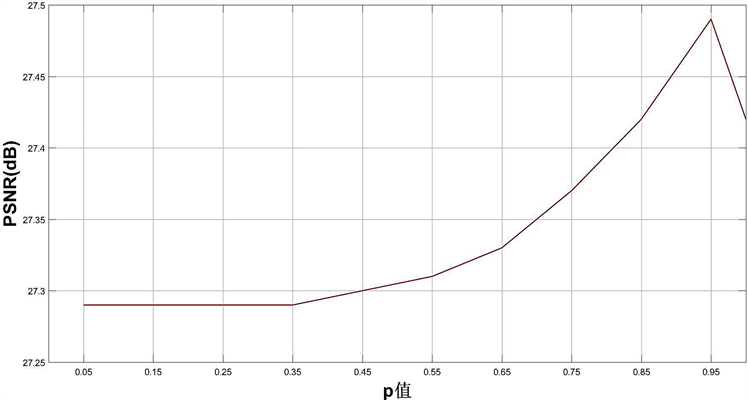Figure 3. The effect of different p values on the mean PSNR of 16 test images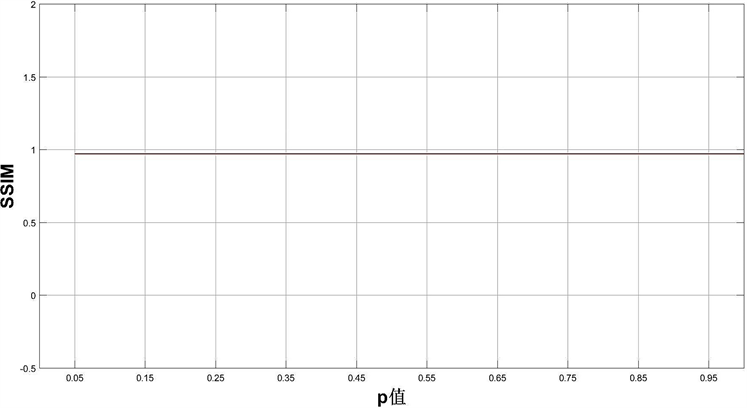Figure 4. The effect of different p values on the mean SSIM of 16 test images

4.2. 仿真实验结果及分析Table 1. Reconstructing results by the above algorithms (PSNR/SSIM)

Continued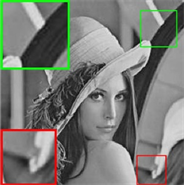(a) 原图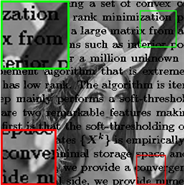(b) 文本覆盖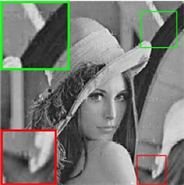(c) IRLS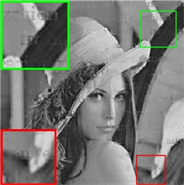(d) PSSV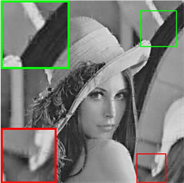(e) LTVNN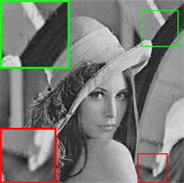(f) SPC-TV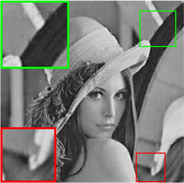(g) SPC-QV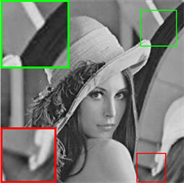(h) WTP-MSTVM

Figure 5. The 16th image is reconstructed by different algorithm. (a) The original image. (b) Text masked image. (c) IRLS: PSNR = 22.14 dB, SSIM = 0.90. (d) PSSV: PSNR = 24.41 dB, SSIM = 0.93. (e) LTVNN: PSNR = 25.33 dB, SSIM = 0.96. (f) SPC-TV: PSNR = 25.33 dB, SSIM = 0.94. (g) SPC-QV: PSNR = 27.07 dB, SSIM = 0.97. (h) WTP-MSTVM: PSNR = 29.20 dB SSIM = 0.98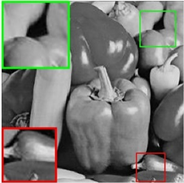(a) 原图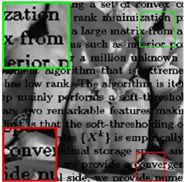(b) 文本覆盖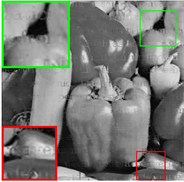(c) IRLS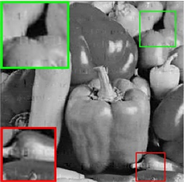(d) PSSV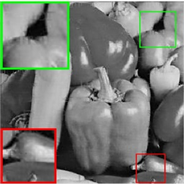(e) LTVNN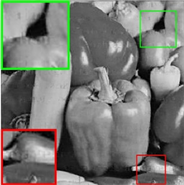(f) SPC-TV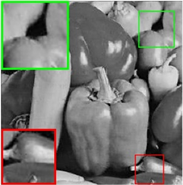(g) SPC-QV(h) WTP-MSTVM

Figure 6. The 14th image is reconstructed by different algorithm. (a) The original image. (b) Text masked image. (c) IRLS: PSNR = 21.43 dB, SSIM = 0.90. (d) PSSV: PSNR = 24.76 dB, SSIM = 0.93. (e) LTVNN: PSNR = 25.19 dB, SSIM = 0.97. (f) SPC-TV: PSNR = 25.13 dB, SSIM = 0.94. (g) SPC-QV: PSNR = 27.20 dB, SSIM = 0.97. (h) WTP-MSTVM: PSNR = 29.76 dB SSIM = 0.99

5. 总结与展望

Matrix Completion Based on Weighted Truncated Schatten-p Norm and Improved Second Order Total Variation[J]. 人工智能与机器人研究, 2019, 08(01): 24-35. https://doi.org/10.12677/AIRR.2019.81004

1. 1. Cabral, R., Del, T., Costeira, P., et al. (2015) Matrix Completion for Weakly-Supervised Multi-Label Image Classification. IEEE Transactions on Pattern Analysis & Machine Intelligence, 37, 121-135. https://doi.org/10.1109/TPAMI.2014.2343234

2. 2. Yong, L., Liu, T., Tao, D., et al. (2015) Multiview Matrix Completion for Multilabel Image Classification. IEEE Transactions on Image Processing, 24, 2355-2368. https://doi.org/10.1109/TIP.2015.2421309

3. 3. Recht, B., Fazel, M. and Parrilo, P.A. (2010) Guaranteed Minimumrank Solu-tions of Linear Matrix Equations via Nuclear Norm Minimization. SIAM Review, 52, 471-501. https://doi.org/10.1137/070697835

4. 4. Nie, F., Huang, H. and Ding, C. (2012) Low Rank Matrix Recovery via Efficient Schatten-p Norm Minimization. Proceedings of the 26th AAAI Conference on Artificial Intelligence, Toronto, Ontario, Canada: AAAI Press, 655-661.

5. 5. Hu, Y., Zhang, D., Ye, J., et al. (2013) Fast and Accurate Matrix Completion via Truncated Nuclear Norm Regularization. IEEE Transactions on Pattern Analysis and Machine Intelligence, 35, 2117-2130. https://doi.org/10.1109/TPAMI.2012.271

6. 6. Oh, T., Tai, Y., Bazin, J., Kim, H. and Kweon, I. (2016) Partial Summinimization of Singular Values in Robust PCA: Algorithm and Applications. IEEE Transactions on Pattern Analysis and Machine Intelligence, 38, 744-758. https://doi.org/10.1109/TPAMI.2015.2465956

7. 7. Komodakis. N. (2006) Image Completion Using Global Optimization. Proceedings of the 2006 IEEE Computer Society Conference on Computer Vision and Pattern Recognition, New York, 17-22 June 2006, 442-452. https://doi.org/10.1109/CVPR.2006.141

8. 8. Keshavan, H. and Montanari, S. (2010) A Matrix Completion from a Few Entries. EEE Transactions on Information Theory, 56, 2980-2998. https://doi.org/10.1109/TIT.2010.2046205

9. 9. Li, W., Zhao, L., Lin, Z., et al. (2015) Non-Local Image Inpainting Using Low-Rank Matrix Completion. Computer Graphics Forum, 34, 111-122. https://doi.org/10.1111/cgf.12521

10. 10. Cand`es, E.J. and Recht, B. (2009) Exact Matrix Completion via Convex Optimization. Foundations of Computational Mathematics, 9, 717-772. https://doi.org/10.1007/s10208-009-9045-5

11. 11. Yu, T., Yan, J., Liu, W. and Li, B. (2018) Incremental Multi-Graph Matching via Diversity and Randomness Based Graph Clustering. Proceedings of the European Conference on Computer Vision (ECCV), Munich, 8-14 September 2018, 139-154. https://doi.org/10.1007/978-3-030-01261-8_9

12. 12. Yu, T. Yan, J., Zhao, J., et al. (2018) Joint Cuts and Matching of Partitions in One Graph. Proceedings of the IEEE Conference on Computer Vision and Pattern Recognition, Salt Lake City, 18-23 June 2018, 705-713. https://doi.org/10.1109/CVPR.2018.00080

13. 13. Koren, Y., Bell, R. and Volinsky, C. (2009) Matrix Factorization Techniques for Recommender Systems. The Computer Journal, 42, 30-37.

14. 14. Gu, S., Xie, Q., Meng, D., et al. (2017) Weighted Nuclear Norm Minimization and Its Applications to Low Level Vision. International Journal of Computer Vision, 121, 183-208. https://doi.org/10.1007/s11263-016-0930-5

15. 15. Xie, Y., Gu, S., Liu, Y., et al. (2016) Weighted Schatten p-Norm Minimization for Image Denoising and Background Subtraction. IEEE Transactions on Image Processing, 25, 4842-4857. https://doi.org/10.1109/TIP.2016.2599290

16. 16. Han, X., Wu, J., Wang, L., Chen, Y. and Shu, H. (2014) Linear Total Variation Approximate Regularized Nuclear Norm Optimization for Matrix Completion. Abstract and Applied Analysis, 2014, Article ID: 765782. https://doi.org/10.1155/2014/765782

17. 17. Wang, W. and Wang, J. (2018) Enhancing Matrix Completion Using a Modified Second-Order Total Variation. Discrete Dynamics in Nature and Society, 2018, Article ID: 2598160. https://doi.org/10.1155/2018/2598160

18. 18. Rodriguez, P. (2013) Total Variation Regularization Algorithms Forimages Corrupted with Different Noise Models: A Review. Journal of Electrical and Computer Engineering, 2013, Article ID: 217021.

19. 19. Papafitsoros, K., Schoenlieb, C.B. and Sengul, B. (2013) Combined First and Second Order Total Variation in Painting Using Split Bregman. Image Processing on Line, 3, 112-136. https://doi.org/10.5201/ipol.2013.40

20. 20. Boyd, S., Parikh, N., Chu, E., Peleato, B. and Eckstein, J. (2011) Distributed Optimization and Statistical Learning via the Alternating Direction Method of Multipliers. Foundations and Trends in Machine Learning, 3, 1-122. https://doi.org/10.1561/2200000016

21. 21. Lin, Z., Liu, R. and Su, Z. (2011) Linearized Alternating Direction Method with Adaptive Penalty for Low-Rank Representation. Proceedings of the 25th Annual Conference on Neural Information Processing Systems, Granada, 12-15 December 2011, 1-7.

22. 22. Benner, P., Li, R.C. and Truhar, N. (2009) On the ADI Method for Sylvester Equations. Journal of Computational and Applied Mathematics, 233, 1035-1045. https://doi.org/10.1016/j.cam.2009.08.108

23. 23. Lai, M.J., Xu, Y.Y. and Yin, W.T. (2013) Improved Iteratively Reweighted Least Squares for Unconstrained Smoothed l𝑞 Minimization. SIAM Journal on Numerical Analysis, 51, 927-957. https://doi.org/10.1137/110840364

24. 24. Wang, Z., Bovik, A.C., Sheikh, H.R. and Simoncelli, E.P. (2004) Image Quality As-sessment: Fromerror Visibility to Structural Similarity. IEEE Transactions on Image Processing, 13, 600-612. https://doi.org/10.1109/TIP.2003.819861

25. 25. Yokota, T., Zhao, Q. and Cichocki, A. (2016) Smooth PARAFAC Decomposi-tion for Tensor Completion. IEEE Transactions on Signal Processing, 64, 5423-5436. https://doi.org/10.1109/TSP.2016.2586759